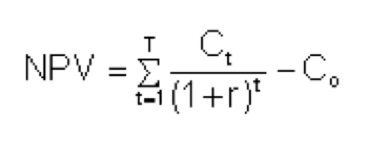# Using internal rate of return (IRR) to measure investment performance

To measure investor’s potential return on an investment, YieldStreet uses the Internal Rate of Return (IRR). Unlike other measurements of an investment’s performance, like Return on Investment (ROI), the IRR can provide a more accurate measure of return by taking into account metrics such as varying cash flow and time passed. IRR is represented as an annualized growth rate, and can give investors an understanding of profit during the lifetime of an investment.

We find that IRR suits YieldStreet investment opportunities because they frequently have varying cash flows from year to year resulting from principal pay-downs (such as debt pay-offs in portfolios of multiple real estate opportunities) and event-based payments (such as case settlements in litigation portfolios).

## Calculating IRR

Simply put, the IRR is the rate earned on every dollar actively invested for each period that it is invested. In technical terms, IRR is an interest rate that makes the net present value (NPV) of all cash flows from a particular investment equal to 0, or in other words, it is the interest rate at which your investment breaks even.

The formula for calculating IRR is as follows:where:

Ct = net cash inflow during the period t

Co= total initial investment costs

r = interest rate, and

t = number of time periods

To calculate IRR, one would set the NPV (net present value) to 0, and solve for r (interest rate).## Breaking down IRR

In order to get a firm grip on how IRR works, let’s break down some of the variables in the formula.

Net Cash Flow During the Period t – This is a net sum of money in and money out during a particular time period. An example of money in would be the interest payment you get from an investment. An example of money out is the initial investment you make at the beginning of the term.

Total Initial Investment Costs – This is the initial investment amount.

Interest Rate – When solved for, this will be the IRR.

NPV (Net Present Value) – The NPV is the net sum of discounted cash flows in an investment, in other words the sum of money that came in (interest payments or principal payments) and money that went out (money spent or invested), while taking into account the passage of time and the yield.

## Assumptions of IRR

For active offerings, the IRR is calculated assuming the investment produces cash flows in the form of interest and principal payments as stated in the loan documents or as projected. The IRR also assumes an annual rate of payment which, when payments occur more frequently, results in a higher IRR than the underlying rate of interest accrual.